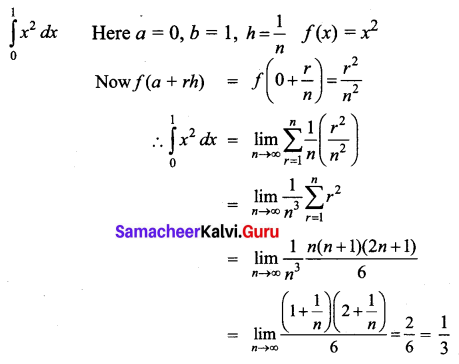# Samacheer Kalvi 12th Business Maths Solutions Chapter 2 Integral Calculus I Ex 2.11

Students can download 12th Business Maths Chapter 2 Integral Calculus I Ex 2.11 Questions and Answers, Samacheer Kalvi 12th Business Maths Book Solutions Guide Pdf helps you to revise the complete Tamilnadu State Board New Syllabus and score more marks in your examinations.

## Tamilnadu Samacheer Kalvi 12th Business Maths Solutions Chapter 2 Integral Calculus I Ex 2.11

Evaluate the following integrals as the limit of the sum:

Question 1.
$$\int_{0}^{1}(x+4) d x$$
Solution: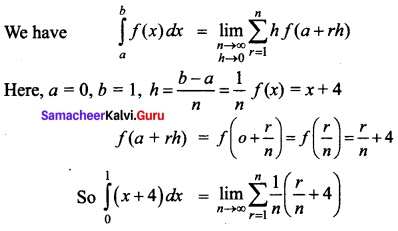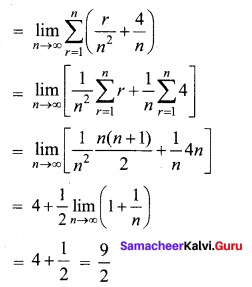Question 2.
$$\int_{1}^{3} x d x$$
Solution: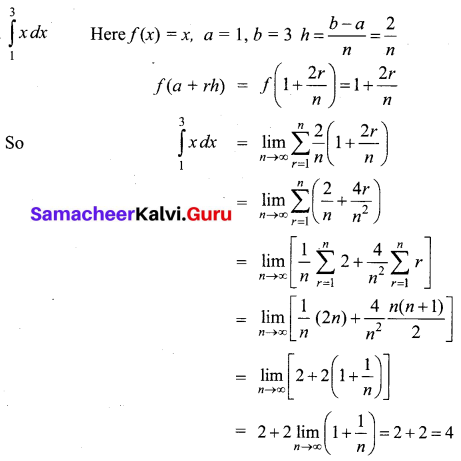Question 3.
$$\int_{1}^{3}(2 x+3) d x$$
Solution: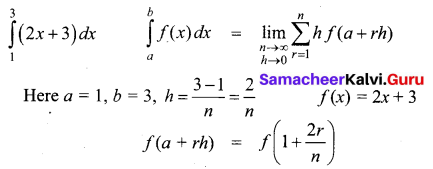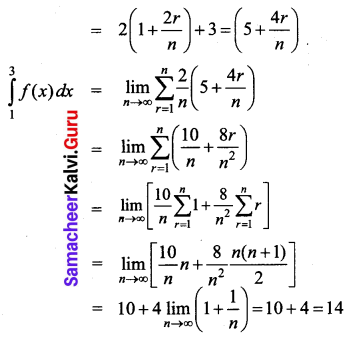Question 4.
$$\int_{0}^{1} x^{2} d x$$
Solution: